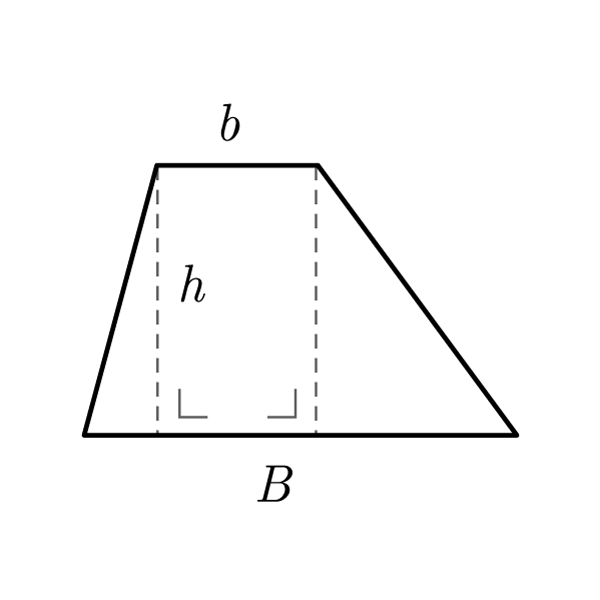# Generic Trapezoid

 Longer Base $$B$$ Shorter Base $$b$$ Oblique Side $$S_{1}$$ Oblique Side $$S_{2}$$ Height $$h$$
$$2p = B + b + S_{1} + S_{2}$$
Perimeter
$$A = \frac{\left(B + b \right) \times h}{2}$$
Area
$$B + b = \frac{2A}{h}$$
Sum of bases
$$h = \frac{2A}{B + b}$$
Height
$$B + b = 2p - S_{1} - S_{2}$$
Sum of bases

## Definition

A trapezoid is a quadrilater with two parallel sides.

## Properties

1. A trapezoid is a four sides polygon with two parallel sides
2. The parallel sides are called bases, while the other two are called oblique sides. In a trapezoid we identify the bases as longer base and shorter base
3. Generic Trapezoid's formulas are valid for any trapezoids## Generic Trapezoid Formulas

Data Formula
Perimeter 2p = B + b + S1 + S2
Area A = [(B + b) × h] / 2
Height h = (2 × A) / (B + b)
Sum of bases B + b = (2 × A) / h
Sum of bases B + b = 2p - S1 - S2